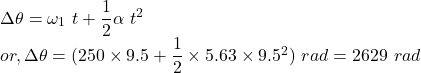## At its lowest setting a centrifuge rotates with an angular speed of ω1 = 250 rad/s. When it is switched to the next higher setting it takes

Question

At its lowest setting a centrifuge rotates with an angular speed of ω1 = 250 rad/s. When it is switched to the next higher setting it takes t = 9.5 s to uniformly accelerate to its final angular speed ω2 = 750 rad/s.

A) Calculate the angular acceleration of the centrifuge α1 in rad/s2 over the time interval t.

B) Calculate the total angular displacement (in radians) of the centrifuge, Δθ, as it accelerates from the initial to the final speed.

in progress 0
5 months 2021-08-31T06:49:34+00:00 1 Answers 12 views 0

Part(a): The angular acceleration is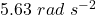.

Part(b): The angular displacement is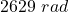.

Explanation:

Part(a):

If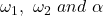be the initial angular speed, final angular speed and angular acceleration  of the centrifuge respectively, then from rotational kinematic equation, we can write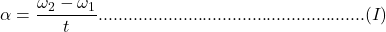where ‘‘ is the time taken by the centrifuge to increase its angular speed.

Given,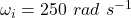,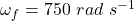and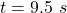. From equation (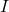), the angular acceleration is given by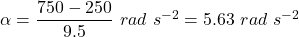Part(b):

Also the angular displacement (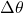) can be written as